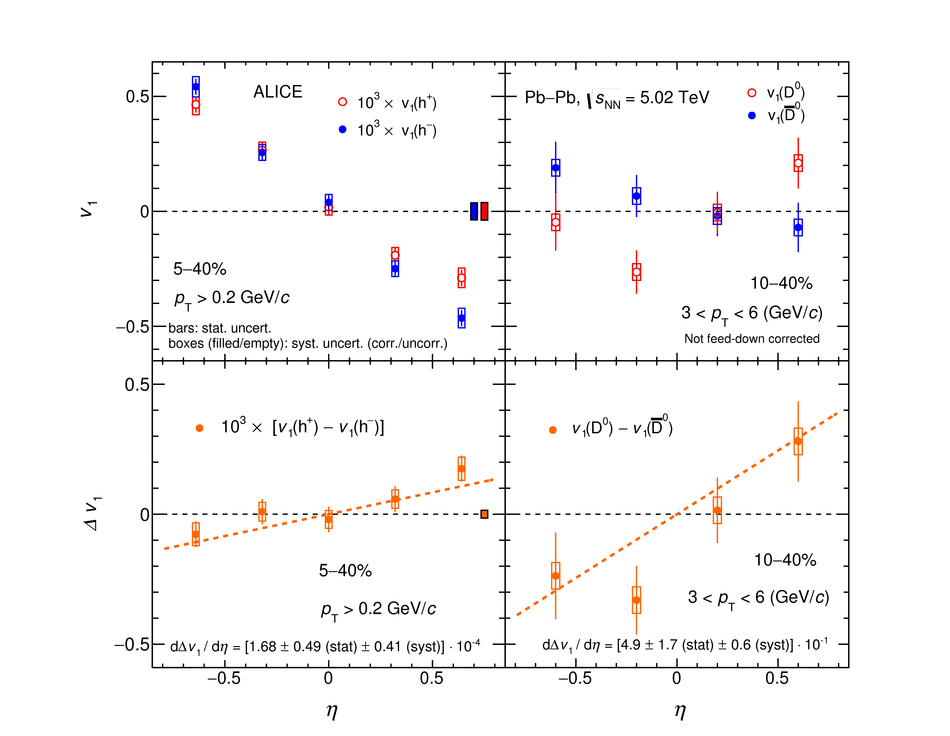# Probing the effects of strong electromagnetic fields with charge-dependent directed flow in Pb-Pb collisions at the LHC

The first measurement at the LHC of charge-dependent directed flow ($v_{1}$) relative to the spectator plane is presented for Pb-Pb collisions at $\sqrt{s_{\rm NN}}$ = 5.02 TeV. Results are reported for charged hadrons and $\rm D^{0}$ mesons for the transverse momentum intervals $p_{\rm T}>0.2$ GeV/$c$ and $3<~p_{\rm T}<~$ 6 GeV/$c$ in the 5-40% and 10-40% centrality classes, respectively. The difference between the positively and negatively charged hadron $v_{1}$ is found to have a positive slope as a function of pseudorapidity $\eta$, ${\rm d}\Delta{v_1}/{\rm d}\eta=$[1.68 $\pm$ 0.49 (stat.) $\pm$ 0.41 (syst.)] $\times 10^{-4}$, with a $2.6\sigma$ significance. The same measurement for $\rm D^{0}$ and $\rm\bar{D}{}^0$ mesons yields a positive value ${\rm d}\Delta{v_1}/{\rm d}\eta$= [4.9 $\pm$ 1.7 (stat.) $\pm$ 0.6 (syst.)]$\times 10^{-1}$, which is about three orders of magnitude larger than the one of the charged hadrons, and is larger than zero with significance of $2.7\sigma$. These measurements can provide new insights into the effects of the strong electromagnetic field and the initial tilt of matter created in non-central heavy-ion collisions on the dynamics of light (u, d, and s) and heavy (c) quarks. The large difference between the observed $\Delta{v_1}$ of charged hadrons and $\rm D^{0}$ mesons may reflect different sensitivity of the charm and light quarks to the early time dynamics of a heavy-ion collision. These observations challenge some of the recent theoretical calculations incorporating effects of the strong electromagnetic field, which predicted a negative and an order of magnitude smaller value of ${\rm d}\Delta{v_1}/{\rm d}\eta$ for both light-flavour and charmed hadrons.

Figures

## Figure 1

 Illustration of the \Dmeson meson $v_{1}$ extraction procedure via a simultaneous fit to the candidate invariant mass distribution (upper panel) and $v_{1}$ (lower panel). Results are for $3 < p_{\rm T} < 6$~GeV/$c$ and $-0.4< \eta< 0$ in the 10--40\% centrality interval in Pb--Pb collisions at $\sqrt{s_{\rm NN}}$ = 5.02~TeV The blue solid lines correspond to the combined signal and background fit functions, while the red dashed lines represent the background contributions Coarse invariant mass intervals are used in the lower panel to reduce statistical fluctuations of the background $v_{1}$.## Figure 2

 Top left: $v_{1}$ of positively (red) and negatively (blue) charged hadrons for the 5--40\% centrality interval in Pb--Pb collisions at $\sqrt{s_{\rm NN}}$ = 5.02 TeV Top right: $v_{1}$ of \Dmeson (red) and \Dbar (blue) for the 10--40\% centrality interval in Pb--Pb collisions at $\sqrt{s_{\rm NN}}$ = 5.02 TeV. Bottom left and right: $\Delta{}v_{1}(h) = v_{1}({h^+}) - v_{1}({h^-})$ and $\Delta{}v_{1}(\rm D) = v_{1}(\Dmeson) - v_{1}(\Dbar)$, respectively Dashed lines represent fits with a linear function